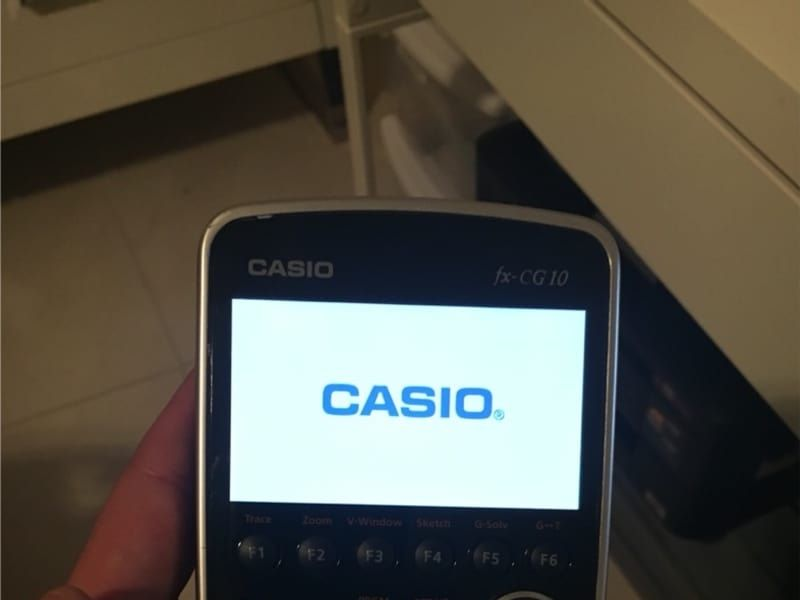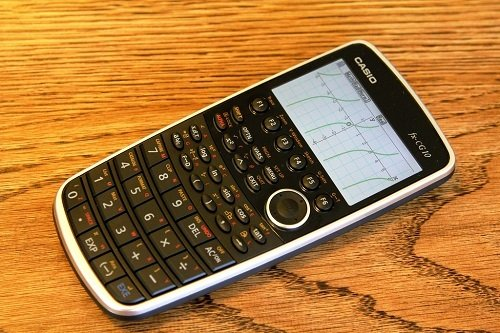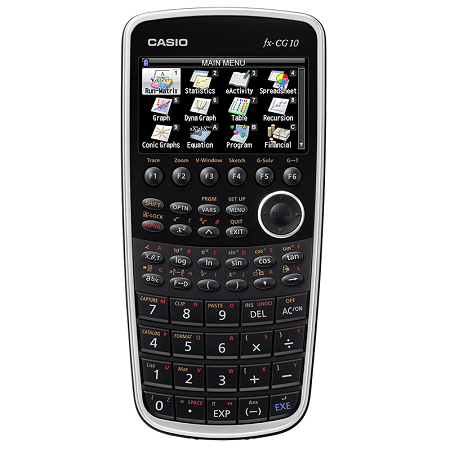# Casio Prizm Graphing Calculator ReviewHow much time would it take for you to computer the answer by hand? On average, students take up to 30 seconds to an hour trying to solve a math problem. By investing in a graphing calculator you can solve the problem virtually instantaneously.

That’s why we’re here to review the Casio Prizm Calculator. It weighs only .5 pounds making it a very compact calculator to have in your bookbag. Continue reading to see if this calculator is worth your money and useful for your next math examination.

The Casio Prizm Calculator is one of the most useful graphing calculators you can find. It features a colorful and bright 216 x 384 pixel display. You can get this on Amazon at a price around \$125.One feature that we like is the calculator’s statistics mode. Shoppers like this mode because their data can be turned into line, bar, and pie charts for further analysis. Get this device if you want to understand statistical concepts and manipulate the data as you see fit.

• “Picture Plot” Technology
• Create graphs out of real life scenes
• Color coded graphs

Another thing that’s worth mentioning is the calculator’s graphing feature. Users found it intuitive and easy to use. The calculator displays the results in a color coded format making it a great choice for students who need to display multiple graphs at once.

With the geometry mode, users are able to draw shapes on the Casio Prizm’s screen. Users can draw lines, circles, triangles, and understand angles. Beginners should look into this device because it helps them understand geometry in an advanced level.

For a more realistic math application, Casio invented a picture plot function. This allows the user to take a picture of a random object in their area and create a mathematical function out of it. For students needing an intense calculator to handle complex equations, this product never fails.

Most consumers disliked the calculator’s learning curve. Beginners took an average of 3 weeks before getting used to the device. We suggest that you look into the instructor’s manual before using this calculator in order to get the most out of it.

Still, the Casio Prizm Calculators is a great engineering calculator and is also good for college level algebra. It has 16MB of memory, making it easy for users to store their data and complete multiple math problems in one setting. Buy it today to maximize your computational skills.

Consider upgrading to a calculator that can handle things like square roots, powers, fractions, or other types of math that you need help with on the ACT.

### Types Of Calculators

Do I need a graphing calculator for a 2 year Epi program, or would a regular old scientific calc work?

#### Basic Calculators

Basic calculators consist of a numerical keypad and can perform simple arithmetic operations. While basic calculators are more superior than their predecessors, they assign only one arithmetic operation or digit to one button.Most basic calculators have a basic display that displays numbers in a row of 10 digits. However, fractions are only able to be represented in decimal notation. Thus, any fractional problem must be converted into decimal form to complete the problem correctly.

All calculators of this type has a basic level of number storage. Most of the basic calculators can store one number in their memory. Get a basic calculator if you only plan on doing simple addition, multiplication, division, and subtraction computations.

#### Scientific Calculators

Scientific Calculators was made a generation before graphing calculators and is still recommended by scientists, mathematicians and teachers. They consist of a single line display, but can display more numbers than your average basic calculator.

The main differences between a graphing calculator and a scientific calculator is that the graphing calculator has the ability to store and write programs, graph data, and display mathematical results on a larger screen.

Scientific calculators can perform mathematical tasks such as: statistics, calculus, standard notation, complex numbers, and much more. In some academic situations where a graphing calculator isn’t allowed, a scientific calculator is the second best option.

#### Graphing Calculators

The first graphing calculator was invented by Casio. Graphing calculators are handheld mathematical devices that are used to solve multiple equations and plot graphs simultaneously. They have the ability to be fully user programmed.

Graphing calculators are the standard for engineering, educational, and scientific purposes. While some might take time getting used to, you’ll have a trustworthy tool that can handle the highest level of mathematical computations with ease.

When looking at a graphing calculator, one of the main differences is their larger display screen. Financial, scientific, and basic calculators have smaller screens which can be difficult for users to see during their exams.

The larger screen allows users to display their data on the x-axis and the y-axis in a easy to read format. Additionally, it shows several lines of text that can be seen at once.

Recent graphing calculators allow you to see your data in 2D, 3D, and in color graphs. Plus, they can aid in creating separate documents of the graphs that you’ve plotted. Graphing calculators have advanced screens to help you analyze data in an accurate manner.

### Compatibilty

Graphing Calculators have Wi-Fi capabilities that help with the logging and evaluating of data from scientific devices. For instance, it can receive data from pH gauges, decibel meters, light meters, electric thermometers, and meterological gauges.Source: casio.com

Also, you’ll want to see if your calculator is compatible with the standardized examination that you plan to take. Fortunately, the Casio Prizm Graphing Calculator can be used on the SAT, ACT, AP, IB, and the PSAT.

## Conclusion

Overall, the Casio Prizm Calculator is definitely worth it if you’re an engineer or a college student. It gives you full range of control over the device and displays your graphing results in a nice color coded display. Buy this product if you want to achieve better results in your classes.

Do you have any questions or comments using this device?